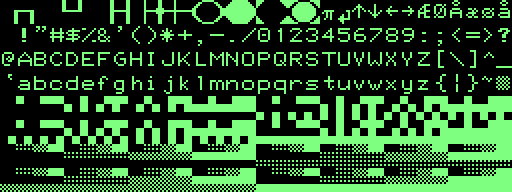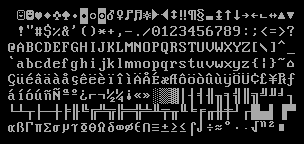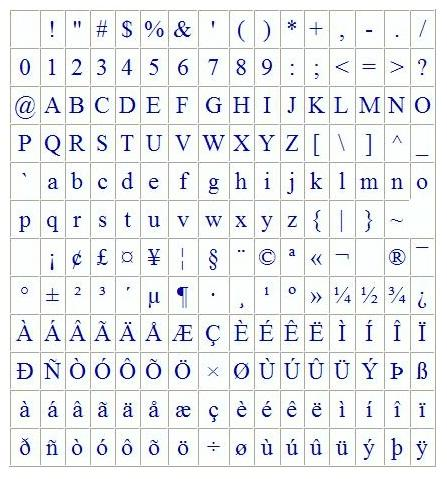Chapter 4 - Data Representation

4
.1  Computers use Binary

Computers have circuits that are either on or off.  Each one of these is a bit.  They are then organized in groups of eight and called bytes.  A computer's word size is when two or more bytes are are addressed and manipulated collectively.  The 8086 had a 16-bit word size.  The 80386 had a 32-bit word size.  Now the x86 CPU's have 64-bit words.

Binary numbers can be tedious to work with.  Hexadecimal provides a shorter way to write binary with one hex digit representing four binary digits.  Programmers write hexadecimal numbers preceded with "0x" such as 0xFF for 255.  The reason for the 0 is so the compiler's parser doesn't mistake a hex number for an identifier which begin with letters.

 Decimal Binary 4-bit Hexadecimal 0 0000 0 1 0001 1 2 0010 2 3 0011 3 4 0100 4 5 0101 5 6 0110 6 7 0111 7 8 1000 8 9 1001 9 10 1010 A 11 1011 B 12 1100 C 13 1101 D 14 1110 E 15 1111 F

As an example, colors are often specified using three bytes.  The bytes can be listed as either decimal or hex and represent the amount of red, green, and blue in the color.  Below are some colors.

 RGB Colors in Hex Red Green Blue Color 00 00 00 black FF FF FF white FF 00 00 red 00 FF 00 green 00 00 FF blue FF FF 00 yellow FF 00 FF magenta 80 80 80 gray FF A5 00 orange

To convert from binary to decimal, you can add up the value of each of the 1's.  Below is an example of how to convert 11010011 to decimal.

 Binary Number 1 1 0 1 0 0 1 1 128+64+16+2+1 = 211 Value of Each Digit 128 64 32 16 8 4 2 1

4.2  Integers

Unsigned integers are stored in memory simply as the binary representation of the number.  A four byte unsigned integer will have a maximum value of approximately 4.3 billion (232).  Below are some examples.

 Unsigned Integers Decimal Binary Hex 2 00000000 00000000 00000000 00000010 00 00 00 02 255 00000000 00000000 00000000 11111111 00 00 00 FF 8,388,608 00000000 10000000 00000000 00000000 00 80 00 00 4,294,967,295 11111111 11111111 11111111 11111111 ‭FF FF FF FF

Signed integers
are can be stored in a variety of methods shown below.

1. Signed Magnitude uses the first bit to represent the sign and the rest of the bits to represent the magnitude of the number.  If the first bit is 0, the number is positive.  If it is 1, the number is negative.  A four byte signed integer now only has 31 bits to hold the number giving a maximum value of +‭2,147,483,647‬ and minimum value of -‭2,147,483,647‬.  One issue is that there are two ways to store the number zero.  Below are some examples.

 Signed Magnitude Integers Decimal Binary Hex 3 00000000 00000000 00000000 00000011 00 00 00 03 -3 10000000 00000000 00000000 00000011 80 00 00 03 0 00000000 00000000 00000000 00000000 00 00 00 00 -0 10000000 00000000 00000000 00000000 80 00 00 00 ‭-65,535 10000000 00000000 11111111 11111111 ‭80 00 FF FF

2. One's Complement flips all the bits to represent negative numbers.  Below are some examples.  Wiki

 One's Complement Integers Decimal Binary Hex 7 00000000 00000000 00000000 00000111 00 00 00 07 -7 11111111 11111111 11111111 11111000 FF FF FF F8 0 00000000 00000000 00000000 00000000 00 00 00 00 -0 11111111 11111111 11111111 11111111 FF FF FF FF ‭255 00000000 00000000 00000000 11111111 ‭00 00 00 FF -255 11111111 11111111 11111111 00000000 FF FF FF 00

One's complement has the advantage of turning subtraction into addition.  To subtract 7 from 255, take the one's complement of 7 and add the two binary numbers.  If there's a high-order carry bit, remove it and add one to the answer.

 One's Complement Subtraction Step Decimal Binary To subract 7 from 255, add them after you make 7 negative using one's complement 255 -7 00000000 00000000 00000000 11111111 11111111 11111111 11111111 11111000 If there's a 1 high-order carry bit, add one to the answer 247 1 00000000 00000000 00000000 11110111‬‬ Answer is 248 248 00000000 00000000 00000000 11111000

2. Two's Complement creates negative numbers by flipping all the bits (like one's complement) and adding 1.  The has the advantage of having only one representation for the number zero.

 Two's Complement Integers Decimal Binary Hex 15 00000000 00000000 00000000 00001111 00 00 00 0F -15 11111111 11111111 11111111 11110001 FF FF FF F1 0 00000000 00000000 00000000 00000000 00 00 00 00 -1 11111111 11111111 11111111 11111111 FF FF FF FF ‭-2 11111111 11111111 11111111 11111110 ‭FF FF FF FE -255 11111111 11111111 11111111 00000001 FF FF FF 01

Two's complement subtraction is the same as one's complement, but there is no need to add one if there's a carry bit.

 Two's Complement Subtraction Step Decimal Binary To subract 2 from 15, add them after you make 2 negative using two's complement 15 -2 00000000 00000000 00000000 00001111 11111111 11111111 11111111 11111110 You can ignore the carry bit 13 1 00000000 00000000 00000000 00001101‬‬

C++ and Java use two's complement to store negative integers.  An int variable can store a number in the range -2,147,483,648 to 2,147,483,647.  A short int variable can store number in the range -32,768 to 32,767.  Negative integers can store one number higher than positive since you add 1 to the number if it's negative.

3. Binary Coded Decimal (BCD) represents each digit of a decimal number using 4 bits.  For example, decimal 1942 is stored as 00011001 01000010.

 1942 Stored as BCD Decimal Digit: 1 9 4 2 4-Bit Binary: 0001 1001 0100 0010‬‬

4.3  Floating-Points

Single precision (32-bit) and double precision (64-bit) floating point numbers are usually represented using the IEEE-754 standard.  Below shows how the bits are used in a single precision floating point.

 Sign Exponent Mantissa 1 bit 8 bits 23 bits

Sign bit - 0 for positive and 1 for negative
Exponent - Use the exponent n where 2n is equal to the nearest number equal or small than the number.  For example, if the number is 17, the exponent will be 4 since 24 = 16.  This number is added to a bias (127 for single precision).  The bias is added because both positive and negative exponents are needed.  Negative exponents are needed for fractions - e.g. 0.25 is 2-2.  Using the bias allows for simpler circuits in the CPU.
Mantissa (also called the significand) - This is the significant portion of the number.  The decimal point is moved so that the first 1 is removed.  For example, if the number is 19 (binary 10011), then the Mantissa will be 0011 with the remaining bits to the right set to 0.  The number of places you move the decimal point is the same as the exponent (before adding the bias).  Fractions can get more complicated and are only introduced here:  0.1 is equal to 1/2, 0.01 is equal to 1/4, 0.001 is equal to 1/8.  Therefore, 4.5 is equal to 100.1

Example 1:  How is 19 stored as a 32-bit floating point?

 Sign Exponent Mantissa 0 10000011 00110000000000000000000 0 for positive 16 is nearest multiple of 2, so the exponent is 4.  This is added to 127 bias. 19 is equal to 10011 The decimal point is moved to the left 4 places leaving 0011 after you drop the 1.

Example 2:  How is 42.5 stored as a 32-bit floating point?

 Sign Exponent Mantissa 0 10000100 01010100000000000000000 0 for positive 32 is nearest multiple of 2, so the exponent is 5.  This is added to 127 bias. 42 is equal to 101010.  For fractions, 0.1 = 1/2, 0.01 = 1/4, 0.001 = 1/8, etc.  Therefore 42.5 is equal to 101010.1 After you move the decimal point 5 places to the left, you have 010101

Here is an online floating point converter for practice.  Here's another site with conversion instructions.

4.4  Characters

To represent characters of the alphabet, a coding system is needed.

EBCDIC was created by IBM in for their System/360 mainframe computers in 1964.  It was compatible with their peripheral equipment such as punch card machines and teletypes.

ASCII (American Standard Code for Information Interchange) was developed in the 1960's by the American Standard's Association and promoted by Bell data services.  It is a descendant of a 5-bit Baudot telegraph code from the 1870's created by Emile Baudot.  The original ASCII code used 7-bits giving 128 different characters and control codes.  The 8th bit could be used as a parity bit.  Parity is used for detecting errors during data transmission.  Traditionally, phone lines could have static causing some bits to be lost.  The parity bit is set to the even or odd depending on the sum of the other bits.  For example, to transmit the letter "a" in 7-bit ASCII you have decimal 97 which is 1100001.  Since the sum of the 1's is odd, the 8th bit is set to 1 (11100001).

 7-bit ASCII Chart 0  NUL 1  SOH - start of heading 2  STX - start of text 3  ETX - end of text 4  EOT - end of transmssn 5  ENQ - enquiry 6  ACK - acknowledge 7  BEL - bell (beep) 8  BS - backspace 9  HT - horizontal tab 10  LF - line feed 11  VT - vertical tab 12  FF - form feed 13  CR - carriage return 14  SO - shift out 15  SI - shift in 16  DLE - data line escape 17  DC1 - device control 1 18  DC2 - device control 2 19  DC3 - device control 3 20  DC4 - device control 4 21  NAK - negative ACK 22  SYN - syncrhonous idle 23  ETB - end transm block 24  CAN - cancel 25  EM - end of medium 26  SUB - substitute 27  ESC - escape 28  FS - file separator 29  GS - group separator 30  RS - record separator 31  US - unit separator 32  space 33    ! 34    " 35    # 36    \$ 37    % 38    & 39    ' 40    ( 41    ) 42    * 43    + 44    , 45    - 46    . 47    / 48    0 49    1 50    2 51    3 52    4 53    5 54    6 55    7 56    8 57    9 58    : 59    ; 60    < 61    = 62    > 63    ? 64    @ 65    A 66    B 67    C 68    D 69    E 70    F 71    G 72    H 73    I 74    J 75    K 76    L 77    M 78    N 79    O 80    P 81    Q 82    R 83    S 84    T 85    U 86    V 87    W 88    X 89    Y 90    Z 91    [ 92    \ 93    ] 94    ^ 95    _ 96    ` 97    a 98    b 99    c 100   d 101   e 102   f 103   g 104   h 105   i 106   j 107   k 108   l 109   m 110   n 111   o 112   p 113   q 114   r 115   s 116   t 117   u 118   v 119   w 120   x 121   y 122   z 123   { 124   | 125   } 126   ~ 127   DEL

The control codes (0 - 31) were for telegraph and teletypes and are no longer used except for 10 (line feed or \n) and 27 (escape).

 Extended ASCII Eventually, the 8th bit is became used to extend the ASCII character set to 256.  The TRS-80 used the extra bit to create blocks for low resolution graphics.  The IBM PC replaced the control characters with symbols like smiley faces and the extended characters were lines for drawing boxes and Greek symbols.  Other companies created their own extended ASCII character set.  It can cause confusion when looking at data or running programs encoded with a different version of the extended ASCII set.TRS-80 extended ASCIIIBM PC DOS extended ASCIILatin-1 extended ASCIIThe most popular extended ASCII character set used is today is referred to as Latin-1.  It has Latin characters for most Western European languages.

Unicode was created to add all the characters used in most of the world's written languages.  Here are two sites where you can see the Unicode character sets:  jgraphix.net  unicode-table.com  The Unicode character set uses 2 bytes giving 65536 different characters.  The UTF-8 encoding specification allows each character to be one to four bytes.  This allows it backwards compatible with ASCII.  UTF-8 is a popular encoding for today's web pages.